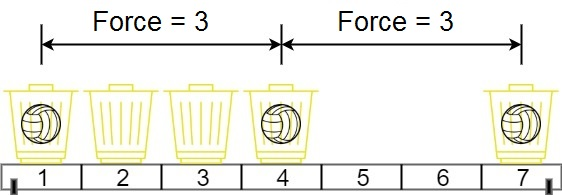Formatted question description: https://leetcode.ca/all/1552.html

# 1552. Magnetic Force Between Two Balls (Medium)

In universe Earth C-137, Rick discovered a special form of magnetic force between two balls if they are put in his new invented basket. Rick has n empty baskets, the ith basket is at position[i], Morty has m balls and needs to distribute the balls into the baskets such that the minimum magnetic force between any two balls is maximum.

Rick stated that magnetic force between two different balls at positions x and y is |x - y|.

Given the integer array position and the integer m. Return the required force.

Example 1:Input: position = [1,2,3,4,7], m = 3
Output: 3
Explanation: Distributing the 3 balls into baskets 1, 4 and 7 will make the magnetic force between ball pairs [3, 3, 6]. The minimum magnetic force is 3. We cannot achieve a larger minimum magnetic force than 3.


Example 2:

Input: position = [5,4,3,2,1,1000000000], m = 2
Output: 999999999
Explanation: We can use baskets 1 and 1000000000.


Constraints:

• n == position.length
• 2 <= n <= 10^5
• 1 <= position[i] <= 10^9
• All integers in position are distinct.
• 2 <= m <= position.length

Related Topics:
Array, Binary Search

## Solution 1. Binary Answer

The range of the answer is [1, A[N-1] - A]. We can use binary search to find the maximum valid distance (i.e. force).

We just need to write a function valid(A, M, m) to check if we can place the m balls with the minimum distance M. This is doable with a linear scan – keep finding the smallest A[i] which is greater than or equal to A[prev] + M where prev is the previously selected busket.

If we can’t find m buskets with M then this M is too large, we need to do R = M - 1, otherwise, we do L = M + 1.

In the end since we are looking for the maximum valid distance, R is the answer.

// Initially
L                                         R
v                                         v
[      valid      ] [       invalid       ]

// Finally
R L
v v
[      valid      ] [       invalid       ]

// OJ: https://leetcode.com/problems/magnetic-force-between-two-balls/

// Time: O(log(max(A)) * N + NlogN)
// Space: O(1)
class Solution {
bool valid(vector<int> &A, int d, int m) {
int prev = -1;
for (int i = 0; i < A.size(); ++i) {
if (prev != -1 && A[i] - prev < d) continue;
if (--m == 0) return true;
prev = A[i];
}
return false;
}
public:
int maxDistance(vector<int>& A, int m) {
sort(begin(A), end(A));
int L = 1, R = A.back() - A;
while (L <= R) {
int M = (L + R) / 2;
if (valid(A, M, m)) L = M + 1;
else R = M - 1;
}
return R;
}
};


Java

class Solution {
public int maxDistance(int[] position, int m) {
TreeSet<Integer> set = new TreeSet<Integer>();
for (int num : position)
Arrays.sort(position);
int length = position.length;
int low = 1, high = position[length - 1] - position;
while (low < high) {
int mid = (high - low + 1) / 2 + low;
if (possible(set, m, mid))
low = mid;
else
high = mid - 1;
}
return low;
}

public boolean possible(TreeSet<Integer> set, int m, int distance) {
int prevPosition = set.first();
m--;
while (m > 0) {
Integer currPosition = set.ceiling(prevPosition + distance);
if (currPosition == null)
return false;
else {
prevPosition = currPosition;
m--;
}
}
return true;
}
}